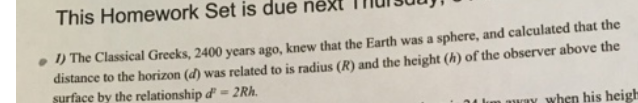### Create an Account

Already have account?

### Forgot Your Password ?

Home / Questions / This Homework Set is due ne . 1) The Classical Greeks, 2400 years ago, knew that the Earth...

# This Homework Set is due ne . 1) The Classical Greeks, 2400 years ago, knew that the Earth was a sphere, and calculated that the distance to the horizon (d) was related to is radius (R) and the height

This Homework Set is due ne . 1) The Classical Greeks, 2400 years ago, knew that the Earth was a sphere, and calculated that the distance to the horizon (d) was related to is radius (R) and the height (h) of the observer above the surface by the relationship d' = 2Rh. My when his heighApr 13 2021 View more View Less

#### Answer (Solved)Subscribe To Get Solution